# NCERT Solutions for Class 7 Maths Chapter 6 The Triangle and its Properties| PDF Download

Here you will find NCERT Solutions for Class 7 Maths Ch 6 The Triangles and its properties which will be helpful in knowing the important points and formulas inside the chapter. It will be useful in passing examinations with flying colours. These NCERT Solutions for Class 7 are accurate and detailed that will increase concentration among students.

Class 7 Maths NCERT Solutions will help you in figuring out the latest marking scheme and devise your own strategy. It will be serve as beneficial tool that can be used to recall various questions any time.1. In APQR, D is the mid-point of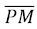is ________
PD is_______
Is QM = MR?QD = DR
∴is altitude.
PD is median.
No, QM (eq) MR as D is the mid-point of QR.

2. Draw rough sketches for the following:
(a) In ΔABC, BE is a median.
(b) In ΔPQR, PQ and PR are altitudes of the triangle.
(c) In ΔXYZ, YL is an altitude in the exterior of the triangle.

(a) Here, BE is a median in ΔABC and AE = EC.(b) Here, PQ and PR are the altitudes of the ΔPQR and RP ⊥ QP.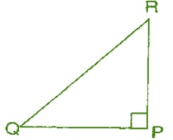(c) YL is an altitude in the exterior of ΔXYZ.3. Verify by drawing a diagram if the median and altitude of a isosceles triangle can be same.

Isosceles triangle means any two sides are same.
Take ΔABC and draw the median when AB = AC.
AL is the median and altitude of the given triangle.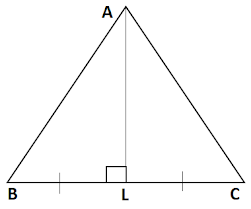Exercise 6.2

1. Find the value of the unknown exterior angle x in the following diagrams: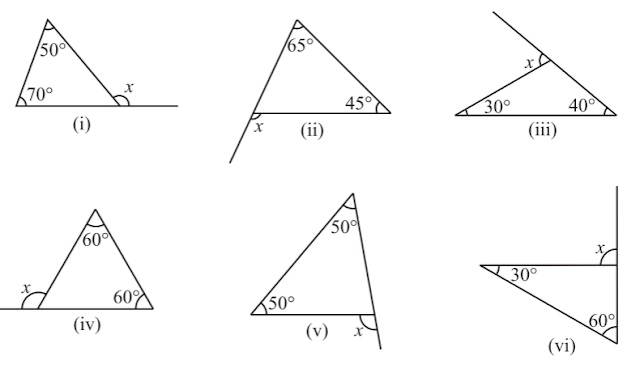(i) x = 50° + 70° = 120°
(ii) x = 65° + 45° = 110°
(iii) x = 30° + 40° = 70°
(iv) x = 60°+60° = 120°
(v) x = 50° + 50° = 100°
(vi) x = 60° + 30° = 90°

2. Find the value of the unknown interior angle x in the following figures:(i)  x + 50° = 115°   ⇒    x = 115° - 50° = 65°
(ii)  70°+ x = 100°    ⇒   x = 100°- 70° =  30°
(iii)  x + 90° = 125°  ⇒  x = 120°- 90° =  35°
(iv)  60°+ x = 120°   ⇒  x = 120°- 60° = 60°
(v)   30° + x = 80°   ⇒   x = 80°- 30° = 50°
(vi)  x + 35°= 75°   ⇒ x = 75°- 35° = 40°

Exercise 6.3

1. Find the value of unknown x in the following diagrams:(i) In ΔABC,
∠ BAC + ∠ ACB + ∠ ABC = 180°  [ By angle sum property of a triangle]
⇒ x + 50°+ 60° = 180°
⇒  x  + 110° = 180°
⇒  x = 180°-110° = 70°

(ii) In ΔPQR,
∠ RPQ + ∠ PQR + ∠ RPQ = 180° [By angle sum property of a triangle]
⇒  90°+30°+ x = 180°
⇒  x+ 120° = 180°
⇒   x= 180°-120°= 60°

(iii) In ΔXYZ,
∠ ZXY + ∠ XYZ + ∠ YZX = 180° [By angle sum property of a triangle]
⇒   30° + 110° + x = 180°
⇒  x + 140° = 180°
⇒ x = 180°-140° = 40°

(iv)  In the given isosceles triangle,
x+x + 50° = 180°   [By angle sum property of a triangle]
⇒  2x+50°= 180°
⇒  2x = 180°- 50°
⇒  2x = 130°
⇒ x = 130°/2 = 65°

(v) In the given equilateral triangle,
x +x+x = 180° [By angle sum property of a triangle]
⇒ 3x = 180°
⇒ x = 180°/3 = 60°

(vi) In the given right angled triangle,
x + 2x+90° = 180°    [By angle sum property of a triangle)
⇒  3x+90° = 180°
⇒  3* = 180°- 90°
⇒ 3x = 90°
⇒ x = 90°/3 = 30°

2. Find the values of the unknowns x and y in the following diagrams: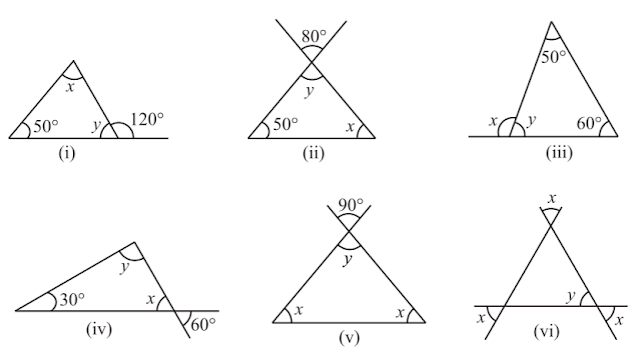(i) 50° + x = 120°    [Exterior angle property of a Δ]
⇒   x = 120°-50° = 70°
Now, 50° + x + y =180°    [Angle sum property of a Δ]
⇒  50° + 70° + y = 180°
⇒  120° + y= 180°
⇒  y = 180° -120° = 60°

(ii) y = 80° ..... (i)    [Vertically opposite angle]
Now, 50° + x + y =180°    [Angle sum property of a Δ]
⇒  50° + 80°+y = 180°    [From equation (i)]
⇒ 130° + y = 180°
⇒ y = 180° -130° = 50°

(iii) 50°+ 60° = x    (Exterior angle property of a Δ]
x = 110°
Now 50° + 60°+ y = 180°    [Angle sum property of a Δ]
⇒  110° + y = 180°
⇒  y = 180° - 110°
⇒  y = 70°

(iv) x = 60° ..... (i) [Vertically opposite angle]
Now, 30° + x + y = 180°    [Angle sum property of a Δ ]
⇒  50° + 60° + y = 180°    [From equation (i)]
⇒ 90° + y = 180°
⇒  y = 180° - 90° = 90°

(v) y = 90°   ....(i)    [Vertically opposite angle]
Now, y + x + x = 180°    [Angle sum property of a Δ]
⇒  90° + 2x = 180°    [From equation (i)]
⇒ 2x = 180°- 90°
⇒  2x = 90°
⇒ x = 90°/2 = 45°

(vi) x = y  ...(i)   [Vertically opposite angle]
Now, x + x + y = 180°    [Angle sum property of a Δ]
⇒ 2x+x = 180°    [From equation (i)]
⇒ 3x = 180°
⇒ x = 180°/3 = 60°

Exercise 6.4

1. Is it possible to have a triangle with the following sides?
(i) 2 cm, 3 cm, 5 cm
(ii) 3 cm, 6 cm, 7 cm
(iii) 6 cm, 3 cm, 2 cm

(i) 2 cm, 3 cm, 5 cm
2 + 3 > 5 No
2 + 5 > 3 Yes
3 + 5 > 2 Yes
This triangle is not possible.

(ii) 3 cm, 6 cm, 7 cm
3 + 6 > 7 Yes
6 + 7 > 3 Yes
3 + 7 > 6 Yes
This triangle is possible.

(iii) 6 cm, 3 cm, 2 cm
6 + 3 > 2 Yes
6 + 2 > 3 Yes
2 + 3 > 6 No
This triangle is not possible.

2. Take any point O in the interior of a triangle PQR. Is:(i) OP + OQ > PQ ?
(ii) OQ + OR > QR?
(iii) OR + OP > RP?

Join OR, OQ and OP.

(i) Is OP + OQ > PQ ?
Yes, POQ form a triangle.

(ii) Is OQ + OR > QR ?
Yes, RQO form a triangle.

(iii) Is OR + OP > RP ?
Yes, ROP form a triangle.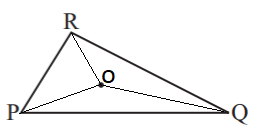3. AM is a median of a triangle ABC. Is AB + BC + CA > 2 AM? (Consider the sides of triangles ΔABM and ΔAMC.)Since, the sum of lengths of any two sides in a triangle should be greater titan the length of third side.
Therefore, In ΔABM, AB + BM > AM ... (i)
In ΔAMC, AC + MC > AM ... (ii)
AB + BM + AC + MC > AM + AM
⇒ AB + AC + (BM + MC) > 2AM
⇒ AB + AC + BC > 2AM
Hence, it is true.

4. ABCD is a quadrilateral. Is AB + BC + CD + DA > AC + BD?Since, the sum of lengths of any two sides in a triangle should be greater than the length of third side.
Therefore, In Δ ABC, AB + BC > AC .........(i)
In ΔDCB, DC + CB > DB  (iii)
Adding equations (i), (ii), (iii) and (iv), we get
AB + BC + AD + DC + DC + CB + AD + AB > AC + AC + DB + DB
⇒ (AB + AB) + (BC + BC) + (AD + AD) + (DC + DC) > 2AC + 2DB
⇒ 2AB + 2BC +2AD + 2DC > 2(AC+DB)
⇒ 2(AB +BC + AD +DC) > 2(AC +DB)
⇒ AB + BC + AD + DC > AC + DB
⇒ AB + BC + CD + DA > AC + DB
Hence, it is true.

5. ABCD is quadrilateral. Is AB + BC + CD + DA < 2 (AC + BD)?

Since, the sum of lengths of any two sides in a triangle should be greater than the length of third side.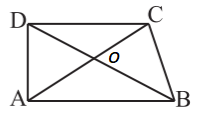Therefore, In A AOB, AB < OA + OB ......... (i)
In A BOC, BC < OB + OC  (ii)
In A COD, CD<OC + OD  (iii)
InAAOD, DA < OD + OA  ....(iv)
Adding equations (i), (ii), (iii) and (iv), we get
AB + BC + CD + DA < OA + OB + OB + OC + OC + OD + OD + OA
⇒ AB + BC + CD + DA < 2OA + 20B + 2OC + 2OD
⇒ AB + BC + CD + DA < 2[(AO + OC) + (DO + OB)]
⇒ AB + BC + CD + DA < 2(AC + BD)
Hence, it is proved.

6. The lengths of two sides of a triangle are 12 cm and 15 cm. Between what two measures should the length of the third side fall?

Since, the sum of lengths of any two sides in a triangle should be greater than the length of third side.
It is given that two sides of triangle are 12 cm and 15 cm.
Therefore, the third side should be less than 12 + 15 = 27 cm.
Also the third side cannot be less than the difference of the two sides.
Therefore, the third side has to be more than 15 – 12 = 3 cm. Hence, the third side could be the length more than 3 cm and less than 27 cm.

Exercise 6.5

1. PQR is a triangle, right angled at P. If PQ = 10 cm and PR = 24 cm, find QR.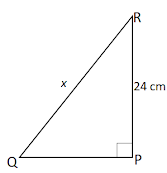Given: PQ = 10 cm, PR = 24 cm
Let QR be x cm.
In right angled triangle QPR,
[Hypotenuse)2 = (Base)2 + (Perpendicular)2   [By Pythagoras theorem]
⇒ (QR)2 = (PQ)2 + (PR]2
⇒ x2 = (10)2 +(24)2
⇒ x2 = 100 + 576 = 676= 26 cm
Thus, the length of QR is 26 cm.

2. ABC is a triangle, right angled at C. If AB = 25 cm and AC = 7 cm, find BC.

Given: AB = 25 cm, AC = 7 cm
Let BC be x cm.
In right angled triangle ACB,
(Hypotenuse)2 = (Base)2 + (Perpendicular)2      [By Pythagoras theorem]
⇒ (AB)2 = (AC)2 + (BC)2
⇒ (25)2 = (7)2+x2
⇒ 625 = 49 + x2
⇒ x2 = 625 - 49 = 576= 24 cm
Thus, the length of BC is 24 cm.

3. A 15 m long ladder reached a window 12 m high from the ground on placing it against a wall at a distance a. Find the distance of the foot of the ladder from the wall.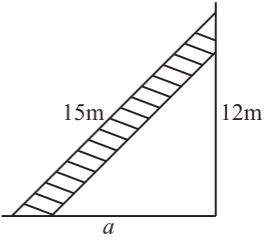Let AC be the ladder and A be the window.
Given: AC = 15 m, AB = 12 m, CB = a m
In right angled triangle ACB,
(Hypotenuse)2 = (Base)2 + (Perpendicular)2    [By Pythagoras theorem]
⇒ (AC)2 = (CB)2 + (AB)2
⇒ (15)2 + (a)2 = (12)2
⇒ 225 = a2 + 144
⇒ a2 = 225 - 144 = 81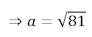= 9 m
Thus, the distance of the foot of the ladder from the wall is 9 m.

4. Which of the following can be the sides of a right triangle?
(i) 2.5 cm, 6.5 cm, 6 cm
(ii) 2 cm, 2 cm, 5 cm
(iii) 1.5 cm, 2 cm, 2.5 cm
In the case of right angled triangles, identify the right angles.

Let us consider, the larger side be the hypotenuse and also using Pythagoras theorem,
(Hypotenuse)2 = (Base)2 + (Perpendicular)2

(i) 2.5 cm, 6.5 cm, 6 cmIn AABC, (AC)2 = (AB)2 + (BC)2
L.H.S. = (6.5)2 = 42.25 cm
R.H.S. = (6)2 + (2.5)2 = 36 + 6.25 = 42.25 cm
Since, L.H.S. = R.H.S.
Therefore, the given sides are of the right angled triangle.
Right angle lies on the opposite to the greater side 6.5 cm, i.e., at B.

(ii) 2 cm, 2 cm, 5 cm
In the given triangle, (5)2 = (2)2 + (2)2
L.H.S. = (5)2 = 25
R.H.S. = (2)2 + (2)2 = 4 + 4 = 8
Since, L.H.S. ≠ R.H.S.
Therefore, the given sides are not of the right angled triangle.

(iii) 1.5 cm, 2 cm, 2.5 cm
In ΔPQR, (PR)2 = (PQ)2 + (RQ)2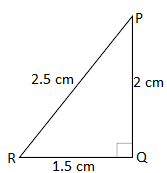L.H.S. = (2 5)2 = 6.25 cm
R.H.S. = (1.5)2 + (2)2 = 2.25 + 4 = 6.25 cm
Since, L.H.S. = R.H.S.
Therefore, the given sides are of the right angled triangle.
Right angle lies on the opposite to the greater side 2.5 cm, i.e., at Q.

5. A tree is broken at a height of 5 m from the ground and its top touches the ground at a distance of 12 m from the base of the tree. Find the original height of the tree.

Let A'CB represents the tree before it broken at the point C and let the top A’ touches the ground at A after it broke. Then ΔABC is a right angled triangle, right angled at B.AB = 12 m and BC = 5 m
Using Pythagoras theorem, In ΔABC
(AC)2 =(AB)2 + (BC)2
⇒ (AC)2 = (12)2 + (5)2
⇒ (AC)2 = 144 + 25
⇒ (AC)2 = 169
⇒ AC = 13 m
Hence, the total height o f the tree = AC + CB 13 + 5 = 18 m.

6. Angles Q and R of a ΔPQR are 25° and 65°
Write which of the following is true:
(i) PQ2 + QR2 = RP2
(ii) PQ2 + RP2 = QR2
(iii) RP2 + QR2 = PQ2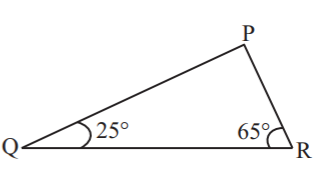In ΔPQR, ∠PQR + ∠QRP + ∠RPQ = 180°      [By Angle sum property of a Δ]
⇒ 25° + 65° + ∠RPQ = 180°
⇒ 90° + ∠RPQ = 180°
⇒ ∠RPQ = 180°- 90° = 90°
Thus, ΔPQR is a right angled triangle, right angled at P.
∴ (Hypotenuse)2 = (Base)2 + (Perpendicular)2    [By Pythagoras theorem]
⇒ (QR)2 = (PR)2 + (QP)2
Hence, Option (ii) is correct.

7. Find the perimeter of the rectangle whose length is 40 cm and a diagonal is 41 cm.

Given diagonal (PR) = 41 cm, length (PQ) = 40 cm
Let breadth (QR) be x cm.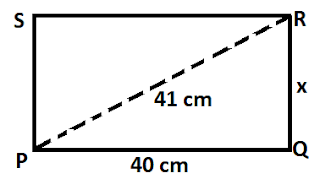Now, in right angled triangle PQR,
(PR)2 = (RQ)2 +(PQ)2      [By Pythagoras theorem]
⇒ (41)2 = x2 + (40)2
⇒ 1681 = x2 + 1600
⇒ x2 = 1681 - 1600
⇒ x2 = 81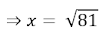= 9 cm
Therefore the breadth of the rectangle is 9 cm,
Perimeter of rectangle - 2(length + breadth)
= 2 [9 + 49)
= 2 × 49 = 98 cm
Hence, the perimeter of the rectangle is 98 cm.

8. The diagonals of a rhombus measure 16 cm and 30 cm. Find its perimeter.

Given: Diagonals AC = 30 cm and DB = 16 cm.
Since the diagonals of the rhombus bisect at right angle to each other.
Therefore, OD = DB/2 = 16/2 = 8 cm
And OC = AC/2 = 30/2 = 15 cm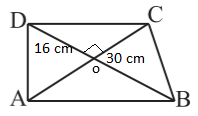Now , In right angle triangle DOC,
(DC)2 =(OD)2 + (OC)2    [By Pythagoras dieorem]
⇒  (DC)2 = (8)2+(15)2
⇒ (DC)2 = 64 + 225 = 289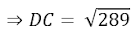= 17 cm
Perimeter of rhombus = 4 x side = 4 x 17 = 68 cm
Thus, die perimeter of rhombus is 68 cm.

## NCERT Solutions for Class 7 Maths Chapter 6 The Triangle and its Properties

These NCERT Solutions are helpful resources that can help you not only cover the entire syllabus but also provide in depth analysis of the topics. A triangle is a closed figure made of three line segments. Every triangle has three sides, three angles, and three vertices. These are known as the parts of a triangle.

• Triangles can be classified based on their sides and angles.
(i) Based on their sides, there are equilateral, isosceles and scalene triangles.
(ii) Based on their angles, there are acute, obtuse and right-angled triangles.

• A triangle is said to be equilateral if each one of its sides is of the same length and each of one its angles measures.

Below we have also provided exercisewise NCERT Solutions that will provide opportunities to learn new things. It can be used to enrich knowledge and make lessons for learners more exciting.

Studyrankers subject matter experts have prepared these step by step NCERT Solutions that will help you get a deeper understanding of various topics and improve your studying habits.

### NCERT Solutions for Class 7 Maths Chapters:

 Chapter 1 Integers Chapter 2 Fractions and Decimals Chapter 3 Data Handling Chapter 4 Simple Equations Chapter 5 Lines and Angles Chapter 7 Congruence of Triangles Chapter 8 Comparing Quantities Chapter 9 Rational Numbers Chapter 10 Practical Geometry Chapter 11 Perimeter and Area Chapter 12 Algebraic Expressions Chapter 13 Exponents and Powers Chapter 14 Symmetry Chapter 15 Visualising Solid Shapes

FAQ on Chapter 6 The Triangle and its Properties

#### How many exercises are there in Chapter 6 The Triangle and its Properties Class 7 Maths NCERT Solutions?

There are total 5 exercises in the Chapter 6 NCERT Solutions which help in revising the chapter properly. These NCERT Solutions are accurate and detailed which will encourage students to learn new topics.

#### What do you mean by right-angled triangle?

A triangle with one of its angles equal to 90° is known as a right-angled triangle. The side opposite the 90° angle is called the hypotenuse, and is the longest side of the triangle.

#### What is Isosceles triangle?

A triangle in which any two sides are equal is called an isosceles triangle. In an isosceles triangle, the angles opposite the equal sides are called the base angles, and they are equal.

#### What do you mean by altitude of a triangle?

The altitude of a triangle is a line segment drawn from a vertex and is perpendicular to the opposite side. A triangle has three altitudes. The altitudes of a triangle are concurrent. The point of concurrence is called the orthocentre, and is denoted by O.